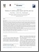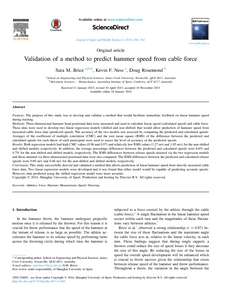# Validation of a method to predict hammer speed from cable force

Brice, Sara M., Ness, Kevin F., and Rosemond, Doug (2015) Validation of a method to predict hammer speed from cable force. Journal of Sport and Health Science, 4 (3). pp. 258-262.Preview
PDF (Published Version) - Published Version

View at Publisher Website: http://dx.doi.org/10.1016/j.jshs.2013.11...

## Abstract

Purpose

The purpose of this study was to develop and validate a method that would facilitate immediate feedback on linear hammer speed during training.

Methods

Three-dimensional hammer head positional data were measured and used to calculate linear speed (calculated speed) and cable force. These data were used to develop two linear regression models (shifted and non-shifted) that would allow prediction of hammer speed from measured cable force data (predicted speed). The accuracy of the two models was assessed by comparing the predicted and calculated speeds. Averages of the coefficient of multiple correlation (CMC) and the root mean square (RMS) of the difference between the predicted and calculated speeds for each throw of each participant were used to assess the level of accuracy of the predicted speeds.

Results

Both regression models had high CMC values (0.96 and 0.97) and relatively low RMS values (1.27 m/s and 1.05 m/s) for the non-shifted and shifted models, respectively. In addition, the average percentage differences between the predicted and calculated speeds were 6.6% and 4.7% for the non-shifted and shifted models, respectively. The RMS differences between release speeds attained via the two regression models and those attained via three-dimensional positional data were also computed. The RMS differences between the predicted and calculated release speeds were 0.69 m/s and 0.46 m/s for the non-shifted and shifted models, respectively.

Conclusion

This study successfully derived and validated a method that allows prediction of linear hammer speed from directly measured cable force data. Two linear regression models were developed and it was found that either model would be capable of predicting accurate speeds. However, data predicted using the shifted regression model were more accurate.

Item ID: 37782 Article (Research - C1) 2213-2961 athletics; force; hammer; measurement; speed; throwing This is an open access article published under the CC-BY-NC-ND 4.0 license. 04 Sep 2015 03:17 11 MEDICAL AND HEALTH SCIENCES > 1106 Human Movement and Sports Science > 110601 Biomechanics @ 100% 97 EXPANDING KNOWLEDGE > 970111 Expanding Knowledge in the Medical and Health Sciences @ 100% Total: 542 Last 12 Months: 1 More Statistics

Actions (Repository Staff Only)Item Control Page